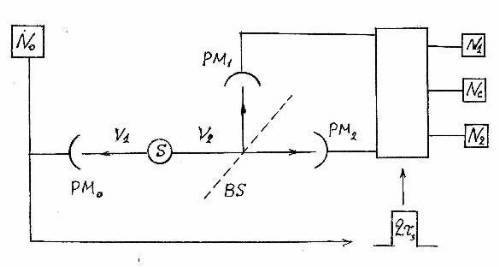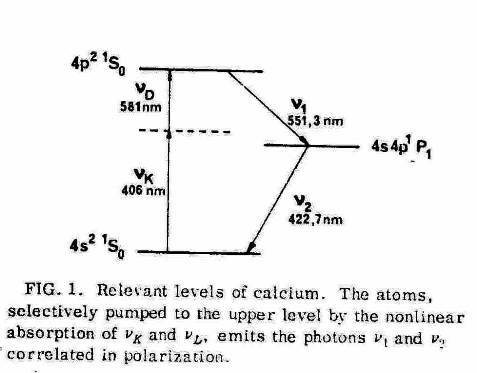THE ERROR OF ALAIN ASPECT. ABOUT ATTEMPT OF THE EXPERIMENTAL PROOF OF PHOTON EXISTENCE.Fig.1. The scheme of installation of Grangier, Roger and Aspect. The signal of detector PM0 from the first photon ν1 opens an electronic gate for the time τ during which photomultipliers PM1 and PM2 are ready to accept the second photon ν2. S – radiation source, N1 – counter of the reflected photons, N2 – counter of the passed photons, Nc – coincidence counter of PM1 and PM2Fig.2
Lev Regelson

Alain Aspect error – in  acceptance of unproved assumption:  in the emission act a "whole" electron always operates. Meamwhile the very existence of  "electron" is a hypothesis which should be proved experimentally as well as "photon" existence. When only small part of an electron cloud participates in radiation act, than the wave train of small intensity will be emitted, and probability of coincidence of signals of such train becomes too small. Thus the main conclusion of Aspect becomes uncorrect.

In 1986 the French physicists Grangier, Roger and Aspect have made the decisive experiment to give the direct answer to a key question of quantum physics – does the light consist of  photons or of wave trains? .

In this experiment (Fig.1) the radiation passed through "beam-splitter":  semitransparent mirror BS which divided it on two equal parts, each registered by it's own detector (PM). If light consists of particles – photons, such particle can or pass through mirror, or be reflected from it: consequently the photon cannot get on both PM at once. If light consists from wave trains, each train will be divided by the mirror on two equal parts which will get on both detectors simultaneously.

For realisation of "one-photon state" the French physicists used cascade emission of atoms of calcium: two photons with different frequencies ν
1 and ν2 are emitted successively during time τs. The first of these photons was used as "triggering" or "starting", the second – as "operating" or "working".

The starting photon caused an impulse on detector PM
0, and this impulse for a while
τ =2τs switched on two other detectors: PM1 (for the photon which has passed through mirror BS) and PM2 (for a photon reflected from a mirror). To distinguish starting and working photons, the filter was put before PM0 passing only frequency ν1, before PM1 and PM2 – the filters passing only frequency ν2. For count of correlations the signals from detectors PM1 and PM2 arrived on the coincidence counter. In case of validity of a photon hypothesis, there should not have place any correlations, in case of absence of photons in wave field of radiation – correlations should be quite frequent.

Counting rates of working signals N
1 and N2 are connected with counting rate of starting signals N0 as:
N1 = ε1 N0
N2 = ε2 N0
where
ε1 and ε2 – registration efficiencies of working  photons, mainly defined by quantum yield of PM and a solid angle of working radiation.
Registration efficiency of starting photons is defined as:

N0 = ε0NF
where N
F - number of photons (or wave trains) in unit of time: the value which cannot be measured directly.

The efficiencies of registration in this experiment, according to an estimation of authors, were equal:

ε1 = ε2 = ε = 0,6х10 (-3)
and accordingly:
N1 =  N2  = N

The theoretical estimation of speed of the account of coincidence NС  from a stream wave trains was defined as:

NС = εε0NF[k +k(2)/2] = ε N [k +k(2)/2]
where
k – an average of light impulses (trains or photons) on detector PM0 during the time
τ =2τs. The second member is defined by casual impositions of light impulses during this interval, therefore for the experiment correctness an observance of condition k <<1 was necessary.

Thus:
NС = k ε                           (1)

Coincidence counting rate observed in experiment was almost 10 times less the value calculated from (1) and could be entirely attributed to casual impositions of starting impulses. Thereby authors make unequivocal conclusion: the light is a stream of the localised particles – photons which cannot be shared on parts.

In the same article  authors inform about interference experiment with the same radiation, consisting of "one-photon states". That the same field of radiation in some experiments manifests corpuscular properties, and in others – wave properties, is accepted as the fact which is not subject to an explanation and this fact has been taken as a basis of all axiomatics of quantum physics.

The authors make a conclusion that they have proved existence of this paradox as well for the radiation structured as the set of one-photon states.

Meanwhile, the experimental proof of the corpuscular nature of the radiation, offered by Alan Aspect with co-authors, contains essential defect to which till now sufficient attention has not been paid.  The fundamental silent assumption has place at the heart of an experiment idea, which in itself requires experimental proof. The question of existence of corpuscular photon demands to pay the attention to the question of existence of corpuscular electron.

Two hypotheses: about localised electron and about a continuous electron field – lead to different conclusions about structure of radiation. If indivisible electron really exists, it wholly participates in each act of emission. By this electron transition the train of electromagnetic field will be emitted with integral intensity always equal to one. Alan Aspect with co-authors have accepted this assumption without any discussion of another hypotheses which however has decisive influence on interpretation of their experiment.

If the electron-corpuscle does not exist, but only continuous electron field, than only a small part of unit electron cloud can transit on upper level. By transition of this part to the lower level, an electromagnetic train of small intensity will be emitted. That will lead to reduction of a theoretical estimation of coincidence probability for one such train.

Thus we should bring the average population of upper level W as a factor to an estimation of  coincidence counting rate (1), namely:

NС = W k ε                                (2)

To estimate a value of parametre W in experiment of Alain Aspect with co-authors, we will address to their earlier articles in which the device of the source of radiation used by them [2, 3] is in detail described. Excitation of levels of cascade transition in atom of calcium was made by means of joint action of two lasers, however there took place the considerable mismatch of frequencies of lasers with frequencies of each separate transition (a Fig. 2 or Fig. 1 in ).  The second step of the cascade had the length of wave of transition 422.7 nanometers whereas the length of a wave of the exciting laser was 406 nanometers. From here we receive a mismatch of frequencies:
∆ω = 2π∆ν = 1,9х10(14)Hz.

The two-level system under the influence of an external field oscillates in such a way that  a part of electron density periodically transits from the lower level to upper one and back (see, e.g. , §5.2). Provided that density of population of the lower level is close to 1, population of upper level is defined as:
W = (ΩR/Ω)(2) sin(2) (Ωt)
where

Ω = √[ΩR(2) + ∆ω(2)]
Under a condition Ω
R <<∆ω expression for population takes a form:

W = (ΩR/∆ω)(2)sin(2)(∆ωt)                                    (3)

Raby frequency Ω
R is defined as:
ΩR = dabE/ћ
where
dab – dipole transition moment, E – amplitude of an external  field.

Intensity of laser radiation in a working zone of a described source can be estimated as   J = 0,3h10
(2)V/cm(2)
and, accordingly, amplitude:
E = (2πJ/с) = 0,5x10(3) V/cm(2)
Raby frequency is thus equal:
ΩR = 1,7х10(9) Hz
Substitution of the values Ω
R and ∆ω into (3) gives an estimation of population density of the upper working level (4s4p¹p1):

W ~ 10(-10)

If electron is an indivisible corpuscle, the density of population W defines probability of its stay at upper level, but in each act of radiation, the whole electron is transiting from upper level unto lower one, with emission of electromagnetic train, naturally "normalized" to 1.
If corpuscular electron does not exist, and there is only a continuous electron field, the value W defines, what part of integrated density of an individual electron state (conditionally accepted for 1), is at upper level. Accordingly, integrated intensity of emitted train will be the same part of unit train. That is equivalent to reduction of total effectiveness, according to (2).
As a result the coincidence counting rate, according to (2), will be:

NС/N = Wkε ~ 0,6х10 (-13)                         (4)

Thus, for acknowledgement or refutation of hypothesis about existence of photons by means of Aspect technique the repetition of experiment is necessary: with condition observance
W ~ 1
It can be reached be means of elimination of a frequencies mismatch, i.e. by selection of lasers with an exact resonance
(∆ω << ΩR) for each of two transitions of the cascade.

Principal conclusion from our reasonings: the question about spatial structure of an electromagnetic field should be considering in parallel with a question about spatial structure of an electron field.

References

1. P.Grangier, G.Roger, A.Aspect. Experimental evidence for a photon anti-correlation effect on a beamsplitter. Europhys. Lett. Vol.1. Pp. 173-179, 1986.
2. A.Aspect, P.Grangier, G.Roger. Experimental Tests of Realistic Local Theories via Bell's Theorem.
Phys. Rev. Lett. Vol. 47, num. 7. Pp. 460-463, 1981.
3. A.Aspect, C.Imbert, G.Roger. Absolute measurement of an atomic cascade rate using a two photon coincidence technique application to the 4p²¹s0 – 4s4p¹p1 – 4s²¹s0 cascade of calcium exited by a two photon absorption.
Optics Comm. Vol. 31, num. 1. Pp. 46-52.
4. M.O.Scully, M.S.Zubairy. Quantum Optics. Cambridge  Univ. Press, 1997.